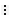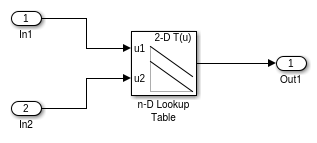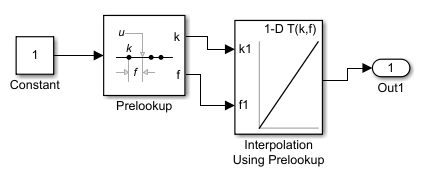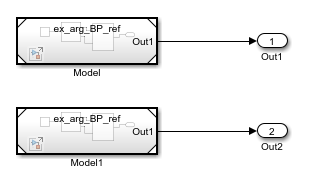## Parameterize Instances of a Reusable Referenced Model

When you reference the same model using multiple Model blocks, you can configure a block parameter to use either the same value or a different value for each instance of the model. For example, you can configure the Gain parameter of a Gain block. To use different values, create and use a model argument to set the value of the block parameter. For some applications, you can reuse a referenced model only if you can configure each instance to use a different value for a block parameter (such as the setpoint of a controller or a filter coefficient).

### Specify a Different Value for Each Instance of a Reusable Model

For a block parameter in a reusable referenced model, to specify a different value for each instance of the model:

1. Create a MATLAB® variable or `Simulink.Parameter` object in the model workspace of the referenced model.

• Use a MATLAB variable for ease of maintenance.

• Use a `Simulink.Parameter` object for greater control over the minimum and maximum value, the data type, and other properties of the model argument.

2. Set the block parameter value by using the variable or parameter object. Optionally, use the same variable or object to set other block parameter values.

3. Configure the variable or object as a model argument by selecting the Argument property.

4. Specify a default value for the variable or `Simulink.Parameter` object. When you simulate this model directly, the block parameters use the value that the variable or object stores in the model workspace. When this model is simulated as a referenced model, a parameter configured as a model argument gets its value from its parent model.

Optionally, you can leave the value for a `Simulink.Parameter` object empty. See Define Model Arguments Without Specifying a Default Value.

5. In each Model block that refers to the reusable model, specify an instance-specific value for the block parameter. The dimensions and data type of the instance-specific value must match that of the model argument definition. If you do not specify a value, the argument uses the last value specified below it in the model hierarchy. In the top model, you can configure the diagnostic configuration parameter No explicit final value for model arguments to generate an error or warning when the topmost Model block that can set the value for a model argument uses this default value instead of providing an explicit value.

6. In intermediate models, in addition to specifying an instance-specific value for the block parameter, you can specify if the parameter can be overridden at the next level of the hierarchy.

### Define Model Arguments Without Specifying a Default Value

If you use a `Simulink.Parameter` object to set the block parameter value, you can compile and simulate the model as a referenced model with the value for the object left empty (Value set to '`[]`') as long as you provide an explicit value somewhere in the parent model reference hierarchy. In this case, you cannot simulate the model directly. When the value is empty, you must provide the Data Type and Dimensions for the object. You can also specify the Min, Max, and Complexity properties. Although you have specified an empty value, Simulink® still synthesizes a Value using the properties you have specified (see `Simulink.Parameter`).

### Combine Multiple Arguments into a Structure

When you configure a model to use multiple model arguments, consider using a structure instead of separate variables in the model workspace. This technique reduces the effort of maintenance when you want to add, rename, or delete arguments. Instead of manually synchronizing the arguments in the model workspace with the argument values in Model blocks, you modify structures by using the Variable Editor or the command prompt.

If you have a Simulink Coder™ license, this technique can also reduce the ROM consumed by the formal parameters of the referenced model functions, such as the output (`step`) function.

To create and use structures to set block parameter values, see Organize Related Block Parameter Definitions in Structures.

### Parameterize a Referenced Model

This example shows how to interactively configure multiple instances of a referenced model to use different values for the same block parameter. For an example that parameterizes a referenced model using only the command prompt, see Parameterize a Referenced Model Programmatically. For an example that involves code generation, see Specify Instance-Specific Parameter Values for Reusable Referenced Model (Simulink Coder).

#### Configure Referenced Model to Use Model Arguments

To configure model arguments for a referenced model, you must create MATLAB variables or `Simulink.Parameter` objects in the model workspace. This example configures `Simulink.Parameter` objects as model arguments without storing a default value in the object and instead relies on the parent model reference hierarchy to provide explicit values. Without a default value, the model cannot be simulated directly and must be simulated as referenced model.

Create a model `ex_model_arg_ref` that contains a Gain block and a Discrete Filter block.To configure the Gain parameter of the Gain block and the Numerator parameter of the Discrete Filter block as model arguments:

1. In the model, on the Modeling tab, click Model Data Editor.

2. In the Model Data Editor, select the Parameters tab.

3. Use the Value column to set the value of the Gain parameter to a variable, for example, `gainArg`.

4. Next to `gainArg`, click the action buttonand select Create.

5. In the Create New Data dialog box, set Value to `Simulink.Parameter` and Location to `Model Workspace`. Click .

6. In the `Simulink.Parameter` property dialog box, set Data Type to `double` and Dimensions to `1`. Leave the value empty (Value set to '`[]`'). Specifying the Min, Max, Complexity values for the object is optional.

7. To configure the object as a model argument, select Argument.

8. Click .

9. Repeat steps 4 through 9 for the Numerator parameter of the Discrete Filter block. In this case, create a `Simulink.Parameter` object named `coeffArg`.

Remember that the model by itself does not compile successfully without values set for the parameter objects.

#### Set Model Argument Values in Parent Model

When you simulate a parent model, each instance of a reusable referenced model uses the parameter values that you specify in the parent model. This example shows how you can expose a model argument as a tunable parameter on the Model block at each level of the model hierarchy.

Create a model `ex_model_arg` that uses multiple instances of the reusable model `ex_model_arg_ref` from the previous example.To set instance-specific values for your model arguments in the model reference hierarchy:

1. In the model, on the Modeling tab, click Model Data Editor.

2. In the Model Data Editor, select the Parameters tab. The Model Data Editor shows four rows that correspond to the instance-specific parameters that you can specify for the two Model blocks.

3. Use the Model Data Editor to set values for the parameters in `Model` and `Model1`. By default, a model argument uses the last value specified below it in the model hierarchy (indicated by the value `<from below>`). Replace the default with the values in this figure.4. To override the value of these parameters at the next level of the model hierarchy, select the check box in the Argument column. By default, the check box is not selected.

You can also configure instance-specific parameters at each Model block. In the block dialog box, select the Instance parameters tab.Tip

Model instance parameters are not supported when ```Use local solver when referencing model``` is enabled in the Solver Pane.

5. Create a model `ex_model_arg_top` that contains a Model block that references `ex_model_arg`.6. In the Model Data Editor, click the button. On the Parameters tab, you can see each instance-specific parameter that is exposed as a tunable parameter in the referenced models. From here, you can create a parameter value set for all instances of the `coeffArg` and `gainArg` parameters in the model hierarchy.By default, each instance uses the last value specified below it in the model hierarchy. In this case, the Model Data Editor displays `<from_below>`. If you select the Argument check box to expose a parameter to a parent model, the Value displays as `<inherited>` to indicate that the runtime value now comes from that parent.

After you update the model diagram, the editor also displays the compiled value for the instance. To navigate to this default value, click the button with the three vertical dots next to the compiled value and select `Navigate to default value from below`. The referenced model where the value was last specified opens in a new tab in the model canvas, and the Model Data Editor highlights the row that contains the block parameter.

Note

You can use a Parameter Writer block to change values of model instance parameters or model workspace variables during simulation. See Initialize and Reset Parameter Values.

#### Group Multiple Model Arguments into Single Structure

You can use structures to reduce the effort of maintenance when you want to add, rename, or delete arguments. With structures, the mathematical functionality of the models is the same.

To replace the parameter values with structures for `ex_model_arg_ref` and `ex_model_arg`, follow these steps:

1. At the command prompt, create a structure. Add one field for each of the parameter objects in the `ex_model_arg_ref` workspace.

```structForInst1.gain = 3.17; structForInst1.coeff = 1.05; ```
2. Store the structure in a `Simulink.Parameter` object.

```structForInst1 = Simulink.Parameter(structForInst1); ```
3. Open the Model Explorer. In the referenced model, `ex_model_arg_ref`, on the Modeling tab, click Model Explorer.

4. Use the Model Explorer to copy the parameter object from the base workspace into the `ex_model_arg_ref` model workspace.

5. In the model workspace, rename `structForInst1` as `structArg`.

6. In the Contents pane, configure `structArg` as the only model argument.7. In the `ex_model_arg_ref` model, in the Model Data Editor Parameters tab, set the value of the Gain parameter to `structArg.gain` and the value of the Numerator parameter to `structArg.coeff`.

8. Save the model.

9. At the command prompt, copy the existing structure in the base workspace as `structForInst2`.

```structForInst2 = copy(structForInst1); ```
10. Set the field values in the two structures by using the same numbers that you used to set the model argument values in the Model blocks.

```structForInst1.Value.gain = 2.98; structForInst1.Value.coeff = 0.98; structForInst2.Value.gain = 3.34; structForInst2.Value.coeff = 1.11; ```
11. In the top model, `ex_model_arg`, use the Model Data Editor to set the argument values as shown in this figure.#### Use Bus Object as Data Type of Structures

You can use a `Simulink.Bus` object as the data type of the structures. The object ensures that the characteristics of the instance-specific structures, such as the names and order of fields, match the characteristics of the structure in the model workspace.

1. At the command prompt, use the function `Simulink.Bus.createObject` to create a `Simulink.Bus` object. The hierarchy of elements in the object matches the hierarchy of the structure fields. The default name of the object is `slBus1`.

```Simulink.Bus.createObject(structForInst1.Value); ```
2. Rename the bus object as `myParamStructType` by copying it.

```myParamStructType = copy(slBus1); ```
3. In the Model Data Editor for `ex_model_arg`, click the Show/refresh additional information button. The Model Data Editor now contains rows that correspond to the parameter objects in the base workspace, `structForInst1` and `structForInst2`.

4. Use the Data Type column to set the data type of `structForInst1` and `structForInst2` to `Bus: myParamStructType`.

5. In the Model Data Editor for `ex_model_arg_ref`, use the Model Data Editor to set the data type of `structArg` to `Bus: myParamStructType`.### Change Model Argument Name or Value

To rename a model argument in the context of the referenced model:

• Find all Model blocks that refer to the model and save the instance-specific parameter values that each block specifies. Use the `get_param` function to query the `InstanceParameters` parameter of each block, which is a structure array. The structure contains four fields: `Name`, `Value`, `Path`, and `Argument`.

You must save the instant-specific parameter values because the renaming operation discards the values in the Model blocks.

• In the Model Data Editor, right-click the variable or object in the model workspace of the referenced model and select Rename All. The renaming operation changes the name of the variable or object and changes references to it throughout the model. For more information, see Create, Edit, and Manage Workspace Variables.

• Reapply the argument values to the Model blocks by using the new name of the argument. To programmatically set argument values in a Model block, see Instance parameters.

### Customize User Interface for Reusable Components

When you design a reusable referenced model for use by other members of a team, you can apply a mask to the entire referenced model. You can then customize the way that your users interact with Model blocks, including setting instance-specific values.

Using this technique also makes it easier to programmatically specify instance-specific values. If you create and use a mask parameter named `gainMask` to programmatically set the value to `0.98` for an instance of the model named `myModelBlock`, your users can use this command at the command prompt:

`set_param('myModelBlock','gainMask','0.98')`

If you apply a mask to the referenced model, the model mask shows only the instance-specific parameters from the direct child model. It does not show instance-specific parameters promoted up from descendant models.

If you do not mask the model, to set the instance-specific value, use the `InstanceParameters` parameter of the block. For more information, see Parameterize a Referenced Model Programmatically.

### Configure Instance-Specific Data for `Simulink.LookupTable` and `Simulink.Breakpoint` Objects

When you use `Simulink.LookupTable` objects to store and configure lookup table data for ASAP2 or AUTOSAR code generation, for example, STD_AXIS or CURVE, you can configure the objects as model arguments. You can also configure a `Simulink.Breakpoint` object that is referenced by a `Simulink.LookupTable` as a model argument. You can then specify unique table data and breakpoint data for each instance of a component.

You can specify the instance-specific value of a `Simulink.LookupTable` argument as a new `Simulink.LookupTable` object in the parent model or as a simple MATLAB structure or array.

Specify an instance-specific value for a `Simulink.LookupTable` object based on the value of the Breakpoints specification property.

• When the Breakpoints specification property is set to `Explicit values` or ```Even spacing```, the instance-specific value of the lookup table object can be:

• A `Simulink.LookupTable` object

• The name of a valid MATLAB structure variable, such as `Model1_LUT2`

• A literal structure expression, such as ```struct(‘Table’, …, ‘BP1’, …, ‘BP2’, …)```

• Other expressions that return a valid structure, such as `Params.Model1.LUT2` or a call to a MATLAB function

• When the Breakpoints specification property is set to `Reference`, the instance-specific value of the `Simulink.LookupTable` object can be:

• A `Simulink.LookupTable` object. In this case, Simulink passes only the Table property of the lookup table object to the child model. This allows you to configure model arguments for `Simulink.LookupTable` objects and `Simulink.Breakpoint` objects independently of each other and reuse these objects as instance parameters for multiple Model blocks.

• A literal numeric array value, such as ```[1 5 7; 2 8 13]```.

• The name of a numeric array variable, such as `Model1_LUT2`.

• Other expressions that return a valid numeric array, such as `Params.Model1.LUT2` or a call to a MATLAB function.

In this case, if you configure the `Simulink.Breakpoint` object that is referenced by the `Simulink.LookupTable` as a model argument, the instance-specific value for the `Simulink.Breakpoint` object can be:

• A `Simulink.Breakpoint` object.

• An array of numeric values that define the breakpoints. The array must match the design definition for the breakpoint object. Simulink synthesizes a `Simulink.Breakpoint` object by using the values in the array.

• The name of a numeric array variable or a field of a structure.

When you specify the instance-specific value of a `Simulink.LookupTable` argument as a structure, these rules apply:

• Each field of the model argument definition must be specified in the structure, and the number of fields and the names of the fields must match. For example, while only the table data from a `Simulink.LookupTable` object of type `Reference` is passed to the child model, you must still specify the breakpoint data in your structure or Simulink reports and error.

• The dimensions of the table and the breakpoint data in the structure must match that of the model argument definition.

• If the data type of a structure field is `double`, the value is cast to the data type of the corresponding model argument field. Otherwise, the value must match the data type of the corresponding model argument field.

You can specify the value of a `Simulink.LookupTable` object as a simple numeric value for any simulation mode and for code generation. For code generation, if you configure the model argument with a storage class of `Auto`, the structure or numeric array variable is not preserved in the generated code. If you set the storage class to any other value, the value of the structure or numeric array is used to initialize the tunable argument in the generated code, similar to other model arguments.

#### Specify Instance-Specific Value of `Simulink.LookupTable` Object

This example shows how to specify the instance-specific value of a `Simulink.LookupTable` argument as a new `Simulink.LookupTable` and as a MATLAB structure.

For an example that parameterizes a referenced model by using lookup tables and the command prompt, see Configure Instance-Specific Data for Lookup Tables Programmatically.

First, create a model that represents a reusable algorithm. Configure a `Simulink.LookupTable` object as a model argument to be used by your model.

1. Create a model `ex_arg_LUT_ref`, which represents a reusable algorithm.2. Using the Model Explorer, add a `Simulink.LookupTable` object in the model workspace. You can use the button. Name the object `LUTArg`.

3. In the Contents pane, for `LUTArg`, select the check box in the Argument column.

4. Set Number of table dimensions to `2`. In the Table and Breakpoints tabular area, specify values for the `Table`, `BP1`, and `BP2` data. For example, configure the table and breakpoint data by entering these values in the MATLAB expression box.

• `Table````[3 4;1 2]```

• `BP1``[1 2]`

• `BP2``[3 4]`

When you simulate or generate code directly from `ex_arg_LUT_ref`, the model uses these values.

5. Under Struct Type definition, set Name to `LUTArg_Type`.

6. Click .

7. In the Contents pane, for `LUTArg`, select the check box in the Argument column.

8. In the referenced model, in the n-D Lookup Table block, set Data specification to ```Lookup table object```. Set Name to `LUTArg`.

9. Save the model.

Next, create a model that uses the reusable algorithm and specify instance-specific values for the `Simulink.LookupTable` object.

1. Create a model `ex_arg_LUT`, which uses the reusable algorithm twice.2. At the command prompt, create a `Simulink.LookupTable` object in the model workspace. Alternatively, you can create the `Simulink.LookupTable` object in a data dictionary or in the base workspace.

```LUTForInst1 = Simulink.LookupTable; ```
3. Specify breakpoint and table data for the object.

```LUTForInst1.Table.Value = [8 7; 6 5]; LUTForInst1.Breakpoints(1).Value = [5 6]; LUTForInst1.Breakpoints(2).Value = [3 4]; ```
4. Specify a structure type name. Match this name to the name specified by the object in the referenced model workspace.

```LUTForInst1.StructTypeInfo.Name = 'LUTArg_Type'; ```
5. Use a structure to create the instance-specific argument value for the second Model block. Specify the breakpoint and table data for the structure.

```StructForInst2.Table = [9 8; 7 7]; StructForInst2.BP1 = [3 4]; StructForInst2.BP2 = [5 6]; ```
6. In the `ex_arg_LUT` model, for model instance `Model`, on the Instance parameters tab, set the value of LUTArg to `LUTForInst1`.

7. For model instance `Model1`, set LUTArg to `StructForInst2`.

One instance of `ex_arg_LUT_ref` uses the table and breakpoint data stored in the `Simulink.LookupTable` object in the model workspace. The other instance uses the table and breakpoint data stored in the structure.

#### Specify Instance-Specific Values for `Simulink.LookupTable` and `Simulink.Breakpoint` Object

This example shows how to specify an instance-specific value of a `Simulink.LookupTable` object as a new `Simulink.LookupTable` object. The example also shows how to specify an instance-specific value for a `Simulink.Breakpoint` object that is referenced by the `Simulink.LookupTable` object.

First, create a model that represents a reusable algorithm. Configure both a `Simulink.Breakpoint` object and the `Simulink.LookupTable` object that references the `Simulink.Breakpoint` object as model arguments.

1. Create a model `ex_arg_BP_ref`, which represents a reusable algorithm.2. Using the Model Explorer, add a `Simulink.LookupTable` object in the model workspace. You can use the button. Name the object `LUTArg`.

3. In the Contents pane, for `LUTArg`, select the check box in the Argument column.

4. Set Number of table dimensions to `1`.

5. Specify the value of the table data as `[1 2]`.

6. Set Breakpoints specification to `Reference`.

7. In the Breakpoints section, set the Names to `BP1`.

8. Add a `Simulink.Breakpoint` object in the model workspace. Name the object `BP1` and specify the value as ```[3 4]```.

9. In the Contents pane, for `BP1`, select the check box in the Argument column.

Next, create a model that uses the reusable algorithm and specify instance-specific values for the `Simulink.LookupTable` object and the `Simulink.Breakpoint` object.

1. Create a model `ex_arg_BP`, which uses the reusable algorithm twice.2. At the command prompt, for model instance `Model`, create a `Simulink.LookupTable` object and a `Simulink.Breakpoint` object in the model workspace. Alternatively, you can create these objects in a data dictionary or the base workspace.

```LUTForInst1 = Simulink.LookupTable; BPForInst1 = Simulink.Breakpoint; ```
3. Specify breakpoint and table data for the objects. You must specify breakpoint information for `LUTForInst1` so that the specification of the instance-specific value matches the model argument specifications. However, only the table data is pushed to the child model.

```LUTForInst1.Table.Value = [7 8]; LUTForInst1.BreakpointsSpecification = 'Reference'; LUTForInst1.Breakpoints = {'BPForInst1'}; BPForInst1.Breakpoints.Value = [5 6]; ```
4. In the `ex_arg_BP` model, for model instance `Model`, on the Instance parameters tab, set the value of LUTArg to `LUTForInst1` and the value of BP1 to `BPForInst1`.

5. For model instance `Model1`, create another `Simulink.LookupTable` object in the model workspace and specify the table and breakpoint data. Alternatively, you can create the `Simulink.LookupTable` object in a data dictionary or in the base workspace.

```LUTForInst2 = Simulink.LookupTable; BPForInst2 = Simulink.Breakpoint; BPForInst2.Breakpoints.Value = [11 12]; LUTForInst2.Table.Value = [9 10]; LUTForInst2.BreakpointsSpecification = 'Reference'; LUTForInst2.Breakpoints = {'BPForInst2'}; ```
6. For this model instance, use an array to specify the instance-specific value for the `Simulink.Breakpoint` object.

```BPArrayForInst2 = [11 12]; ```
7. In the `ex_arg_BP` model, for model instance `Model1`, on the Instance parameters tab, set the value of LUTArg to `LUTForInst2`. For this model instance, set the value of BP1 to the array `BPArrayForInst2`.